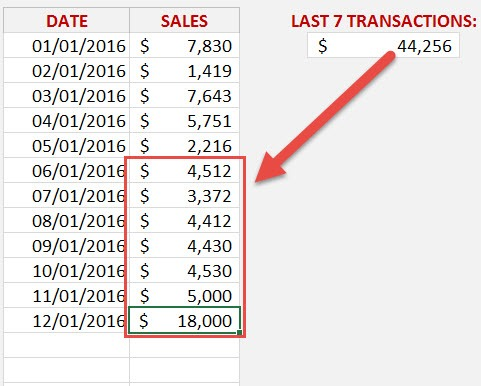What does it do?

It returns a reference to a range, from a starting point to a specified number of rows, columns, height and width of cells

Formula breakdown:

=OFFSET(reference, rows, columns, [height], [width])

What it means:

=OFFSET(start in this cell, go up/down a number of rows, go left/right a number of columns, height of range, width of range)

The OFFSET function in Excel is one of the Lookup functions and is great if you want to reference a range of cells and use that reference to do a calculation.

It is often used when you need to reference a range that is moving or resizing.  For example, if we have daily sales going down one row per day, then we can Sum the last X transactions.

This is what I am going to show you in this tutorial below:

STEP 1: We need to enter the Sum function as we are going to Sum the last 7 transactions

(We can also add the Average function if we want to show the Average of the last 7 transactions):

## =SUM

STEP 2: We need to enter the Offset function so we can reference the range that we want to Sum:

## =SUM(OFFSET

STEP 3: 1st Offset argument – Where do we want to to start our reference?

This is at the start of the Daily Sales list:

## =SUM(OFFSET(D11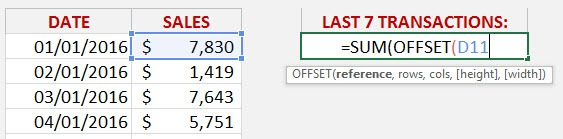STEP 4: 2nd Offset argument – How many Rows down do we want to go?

This is where the COUNTA function is used to count how many transactions we have in total and go down to the last cell in our list.

So as we are starting at cell D11, we are going to go down 11 cells ( COUNTA(D11:D100) ) and end up after the last cell with a value.

NB: It is always a good idea to enter an ending range that is more than your last data cell.  That way as your new data get entered, we will be sure to capture it!

## =SUM(OFFSET(D11, COUNTA(D11:D100)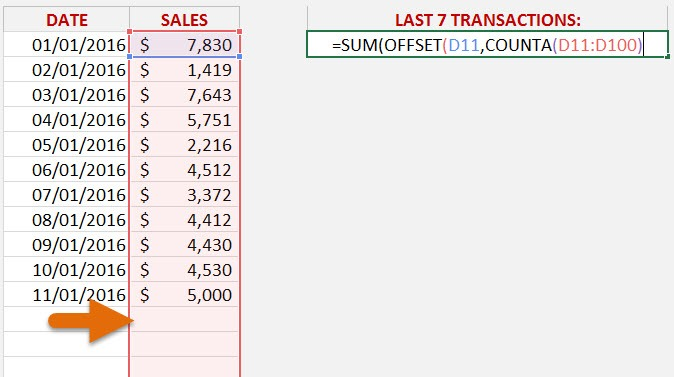STEP 5: How many of the last sales transactions do we want to Sum?  7

So we need to take away 7 from the COUNTA formula which means that our Offset function will go up by 7 cells:

## =SUM(OFFSET(D11, COUNTA(D11:D100)-7,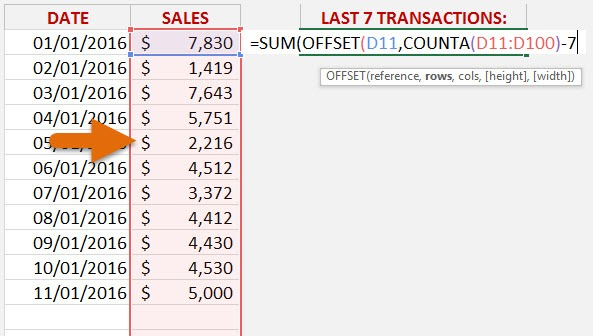STEP 6: 3rd Offset argument – How many Columns to the right/left do we want to move?

We do not want to move to any Columns, so we simply enter 0

## =SUM(OFFSET(D11, COUNTA(D11:D100)-7,0,

STEP 7: 4th Offset argument – How High do we want our referenced data to be?

Since we want to Sum 7 transactions, then we need the [height] to be 7 cells high:

## =SUM(OFFSET(D11, COUNTA(D11:D100)-7,0,7,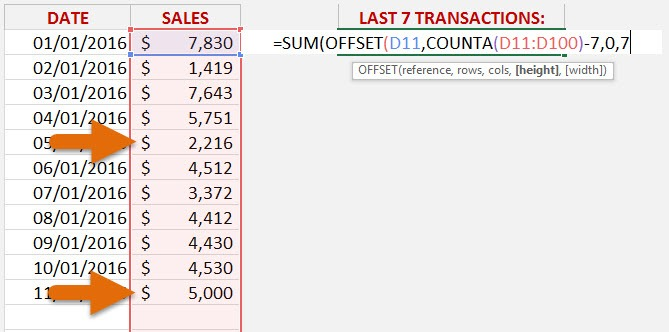STEP 8: 5th Offset argument – How Wide do we want our referenced data to be?

We want to reference 1 Column only, so we need to enter 1 for the [width]

## =SUM(OFFSET(D11, COUNTA(D11:D100)-7,0,7,1))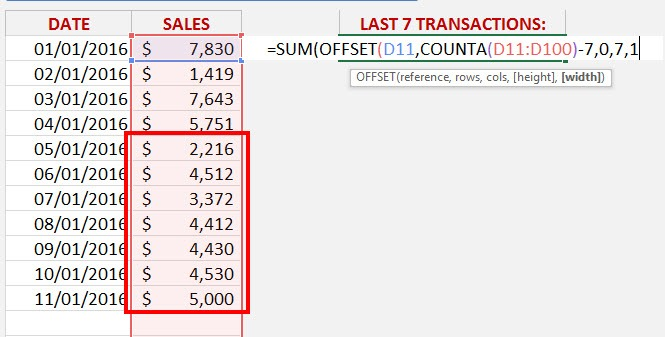As we can see the Sum of the last 7 transactions from our example is \$28,472.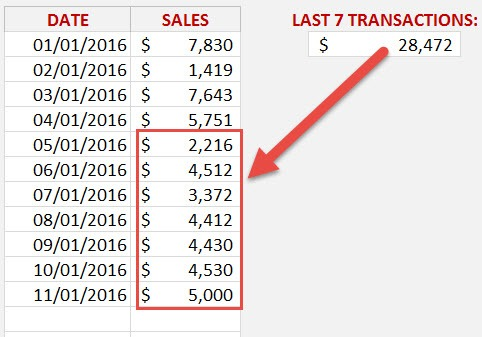If we add more transactions at the bottom of our Sales list then it it automatically return us the last 7 transactions, without the need to update the formula 🙂